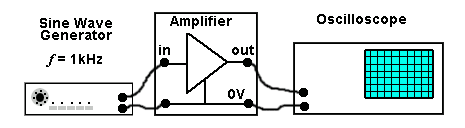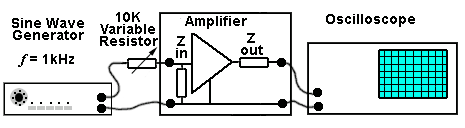# Testing the Amplifier

• After studying this section, you should be able to:
• Test the amplifier operation using a multimeter, signal generator and oscilloscope for:
•   • Gain, Bandwidth, Input and Output Impedance.
• Understand the importance of individual component values relating to:
•   • Gain, Bandwidth, and Distortion.

## Part 3. Testing the amplifier under signal conditions.

Record your results in Part 3 of the Amplifier Design Record sheets.

### 1. Initial check.

Visually double check the circuit, especially the capacitor connections (see warning in Part 2). Switch on and re-check the transistor voltages to make sure the circuit is operating as predicted.

### 2. Gain (Voltage Amplification Av).

Gain can be measured using the set up shown in Fig 2.3.1. The generator is set to a mid-band frequency of 1kHz and a small amplitude sine wave signal applied to the amplifier input. With the oscilloscope attached to the output terminals, the input signal is adjusted to give a large amplitude output signal that still has an undistorted waveform. The peak-to-peak amplitude of the output signal is measured and then the oscilloscope probes are transferred to measure the input. The two values are compared, and the Small Signal Voltage Amplification (Av) is calculated using the formula on the Amplifier Design Record sheets.

Av is simply the ratio of output to input, so does not have any units.### Input Impedance.

Since the input of the amplifier will be mainly resistive at frequencies around or below 1kHz the input impedance Zin can be represented in the diagram of the amplifier (Fig. 2.3.2) as a resistor across the input terminals. To find the value of Zin (at 1kHz) a variable resistor of about 10K ohms (a larger value than the amplifier input impedance is expected to be), or a decade resistance box can be connected between the generator and the amplifier input as shown in Fig. 2.3.2.### Fig. 2.3.2 Measuring the Input Impedance Zin

Initially the variable resistor is set to zero ohms and the generator is adjusted to give a large undistorted display on the oscilloscope. The amplitude of the display on the oscilloscope should be adjusted to fit exactly between an even number of the horizontal graticule markings.

The variable resistor is now adjusted until the peak-to-peak of the output wave is exactly half its original value. Disconnect the variable resistor taking care not to disturb the slider position, and measure its resistance value with the multi-meter. As the variable resistor and the input impedance must both be the same value to give 50% of the amplitude across each, the resistance value of the variable resistor is therefore the same value as Zin.

### Output Impedance Zout

The output of the amplifier is developed across the load resistor RL so this resistor is effectively the output resistance (and approximately the output impedance Zout at 1kHz) of the amplifier. The output coupling capacitor C2 (see Fig. 2.2.1) will not have a significant effect on Zout as it will have a very low reactance at 1kHz.

C4 (when fitted later), will effectively be in parallel with RL but as it will have a very high reactance over most of the amplifier’s bandwidth, it will not greatly affect the output impedance Zout at 1kHz.

### Bandwidth.

Checking the bandwidth of the amplifier requires the same equipment set up as in Fig. 2.3.1 but this time the frequency of the input will be varied.### Fig. 2.3.3 Measuring the Amplifier Bandwidth

a.) Initially set the generator frequency to 1kHz and adjust the generator amplitude and the oscilloscope controls to view a large, undistorted waveform, adjust the amplitude of the waveform to fit exactly between an even number of horizontal graticule lines on the oscilloscope display.

b.) Calculate the −3dB level by multiplying the Vpp value observed in a.) by 0.707.

c.) Without altering the generator amplitude, reduce the frequency of the input wave and observe its VPP amplitude on the oscilloscope. Keep reducing the frequency until the amplitude of the output wave falls to 0.707 of that observed at 1kHz. This is the low frequency −3dB limit of the bandwidth.

d.) Increase the frequency past 1kHz until the output VPP again falls to 0.707 of the 1kHz value (this may be up to 100kHz or even be higher). This frequency is the high frequency −3dB limit of the bandwidth.

It is quite probable that the tests will show that the amplifier bandwidth will not conform to a nice 20Hz to 20kHz specification, or that there may be variations in maximum gain over the frequency range. This is not the ideal situation for a good audio amplifier, so the design may need improving as described in Amplifiers module 2.4.

Top of Page